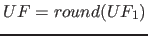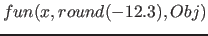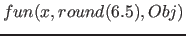Next: Delay Up: Real subfunctions Previous: Absolute value   Contents   Index

#### Round

This function rounds the value of the subfunction to an integer value. For the rounding, the decimal digit befor the point remains the same, if the first decimal digit after the point is between 0 to 4, otherwise (from 5 to 9) the number of the decimal befor the point is increased by one for positive numbers and decreases by one for negative numbers.

Syntax:Examples:

•; the returned value is -12
•; the returned value is 7
•; the returned value is -5

Betti Österholz 2013-02-13